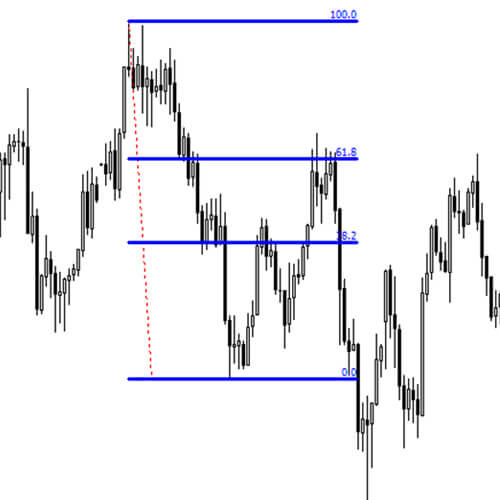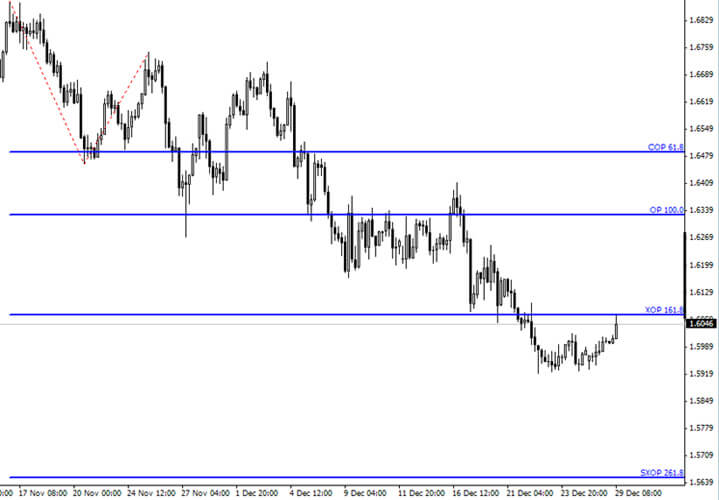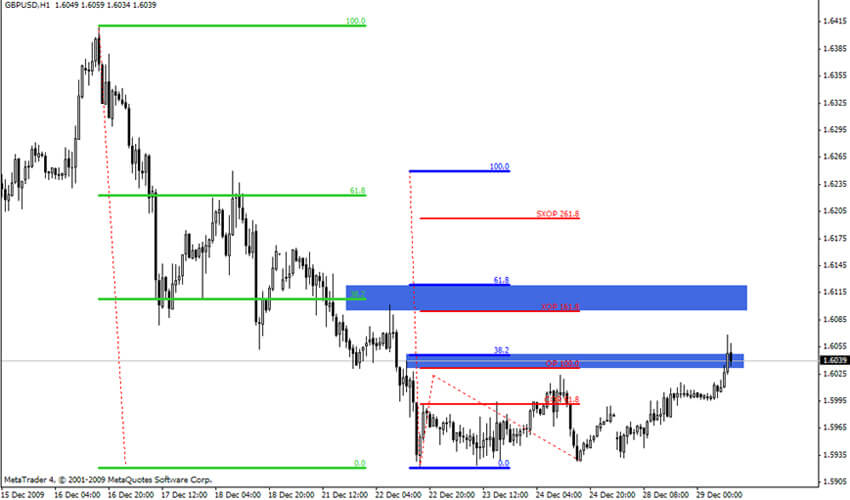Joe Dinapoli Style: How to Use Fibonacci Levels

# Joe Dinapoli Style: How to Use Fibonacci Levels

Fibonacci retracements and expansions in Joe DiNapoli style, or DiNapoli levels, should be considered as the whole system. None element can be viewed separately.

Below is a guide to the Fibonacci levels use in DiNapoli trading.

Of all the available retracement levels (.236, .382, .50, .618, .764) J. DiNapoli has chosen only 2: .382 and .618. In my opinion, this choice is such because the two levels are used for entries, and it does not matter which one you enter at – 61.8% or 76.4% - in both cases your protective stop will rest below/above the beginning of the wave (or the reaction). You can see how the corrective fib-nodes look like on Fig.1. And being familiar with the DiNapoli entry techniques, you know that these two levels are more than enough.

Figure 1. Corrective fib-nodesThe number of expansion levels is also small. The author uses only 3 of them, and one more – the super expanded objective point – was added later by other traders who used his techniques.

1. COP - contracted objective point = .618 of the length of wave A measured from the end of a corrective wave B;

2. ОР - objective point = 100% of the length of a wave A measured from the end of the wave B;

3. XOP - expanded objective point = 1.618 of the wave A measured from B;

4. SXOP - super expanded objective point = 2.618 of the wave A measured from B.

The obvious conclusion to applying these particular expansions is that the length of the corrective wave also plays its role: all the measurements start from the end of the corrective wave B. I believe this is a very logical and reasonable technique.Neophytes to currency trading often calculate expansions and retracements of the waves whose role and place is vaguely understood. To bring some order to the calculations, Joe DiNapoli introduces the "reaction number" term, which gives its specific corrective Fib-nodes. Below is a screenshot that shows reaction numbers and their respective Fibonacci expansions. Thanks to the reaction numbers, you will know what each expansion point means and where it derives from. Also you can see areas of "dating", where expansions off various reaction numbers fall into one area, at least approximately.The last screenshot shows all the actual Fibonacci retracements, expansions and the areas where they intersect giving strong supports and resistances.Can't speak right now?